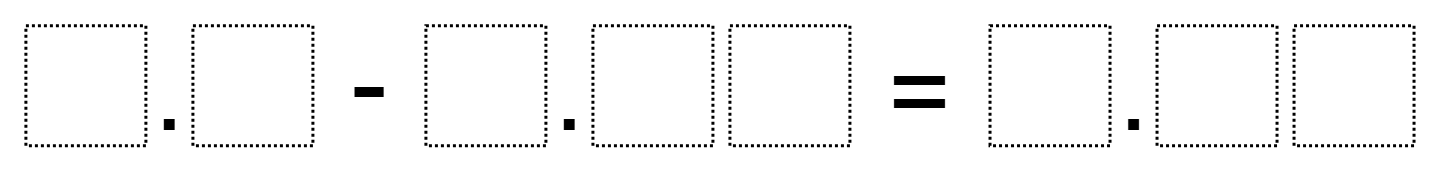# Subtracting Decimals 2

Directions: Using the digits 1-9, at most once each, fill in the boxes to make a true statement.### Hint

Where do you find it most manageable to begin placing digits?   Think about what digit will end up in the hundredths place of the difference based on the digit you use in the hundredths place of the subtrahend.

There are multiple solutions. Three solutions are 9.5-7.34=2.16; 8.7 – 3.29 = 5.41; 9.1-5.24=3.86

## Volume of Rectangular Prisms 2

Directions: Using the digits 1 through 9, at most one time each, place a digit …

1.2.Sheldon Enrichment

Here is the answer we came up with at Greene County Middle School

3.Sheldon Enrichment

9.8-4.63=5.17

4.5.90 – 3.74 = 2.16

5.666 + 666 = you will be dead

6.9.80 – 5.67 = 4.13

7.9.0-2.50=6.50

8.8.9 – 7.56 = 1.34

9.9.4-6.83=2.57